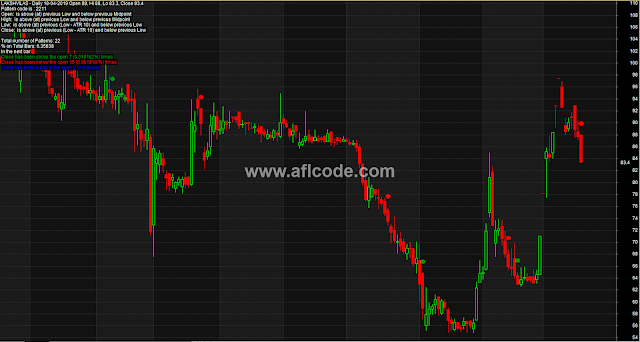### Market Commentary With Multiple IndicatorsMarket Commentary With Multiple Indicators
```
//www.aflcode.com

function msPattPos( element )
{
Value1 = Ref( H, -1 );
Value2 = Ref( L, -1 );
Value3 = ( Value1 + Value2 )/2;
Dist = Ref( ATR( 10 ), -1 );
Value4 = Value1 + Dist;
Value5 = Value2 - Dist;

result = IIf( element < Value5, 0,
IIf( element < Value2, 1,
IIf( element < Value3, 2,
IIf( element < Value1, 3,
IIf( element < Value4, 4,
5 ) ) ) ) );

return result;
}

function msPattToText( patt )
{
result = WriteIf( patt == 0, " is below previous (Low - ATR 10) ",
WriteIf( patt == 1, " is above (at) previous (Low - ATR 10) and below previous Low ",
WriteIf( patt == 2, " is above (at) previous Low and below previous Midpoint ",
WriteIf( patt == 3, " is above (at) previous Midpoint and below previous High ",
WriteIf( patt == 4, " is above (at) previous High and below (High + ATR 10) ",
" is above (at) previous (High + ATR(10) " ) ) ) ) );

return result;
}

function msRecognize()
{
return 1000 * msPattPos( Open ) + 100 * msPattPos( High ) + 10 * msPattPos( Low ) + msPattPos( Close );
}

function msPatternDescription( patt )
{
return "Open: " + msPattToText( round( ( patt / 1000 ) % 10 ) ) + "\n" +
"High: " + msPattToText( round( ( patt / 100 ) % 10 ) ) + "\n" +
"Low: " + msPattToText( round( ( patt / 10 ) % 10 ) ) + "\n" +
"Close: " + msPattToText( round( patt % 10 ) );
}

patts = msRecognize();

// by default use pattern occuring at selected bar
DesiredPattern = SelectedValue( patts );

// if you want manual-entry of pattern code from parameter dialog
// then uncomment the line below
//DesiredPattern=Param("Pattern to look for", 3434, 0, 5555, 0 );

Title = StrFormat("{{NAME}} - {{INTERVAL}} {{DATE}} Open %g, Hi %g, Lo %g, Close %g", O, H, L, C);
Title = Title + "\nPattern code is : " + DesiredPattern + "\n" +
msPatternDescription( DesiredPattern );

Plot( C, "Price", ParamColor("Color", colorBlack ), styleNoTitle | ParamStyle("Style") | GetPriceStyle() );

PattCloseAbove = DesiredPattern == patts AND Ref( Close > Open, 1 );
PattCloseBelow = DesiredPattern == patts AND Ref( Close < Open, 1 );

PlotShapes( ( DesiredPattern == patts ) * shapeCircle ,
IIf( PattCloseAbove, colorGreen,
IIf( PattCloseBelow, colorRed, colorBlue ) ),
0, High, 30 );

NumPatterns = LastValue( Cum( DesiredPattern == patts ) );

NumPattCloseAbove = LastValue( Cum( PattCloseAbove ) );
NumPattCloseBelow = LastValue( Cum( PattCloseBelow ) );
NumPattCloseEqual = NumPatterns - NumPattCloseAbove - NumPattCloseBelow;

Title = Title +
"\n\nTotal number of Patterns: " + NumPatterns +
"\n% on Total Bars: " + 100 * NumPatterns/BarCount +
"\nIn the next bar\n" +
EncodeColor(colorGreen) +
"Close has been above the open " + NumPattCloseAbove +
" (" + NumPattCloseAbove / NumPatterns + "%) times\n" +
EncodeColor(colorRed) +
"Close has been below the open " + NumPattCloseBelow + " (" +
NumPattCloseBelow / NumPatterns +" %) times\n" +
EncodeColor(colorBlue) +
"Close has been equal to the open " + NumPattCloseEqual + " (" +
NumPattCloseEqual / NumPatterns +" %)times";

_SECTION_END();
```
Previous
Next Post »